# Introduction

This tutorial provides an overview of how to perform popular analysis methods and visualizations commonly applied to tracking data. Examples range from the simple analysis of speed or other measures on single tracks, to the application of step-based or staggered metrics, to the generation of autocorrelation plots and performing angle analyses.

# Datasets

library( celltrackR )
library( ggplot2 )


The package contains a dataset of T cells imaged in a mouse peripheral lymph node using two photon microscopy. We will here use this dataset as an example of how to perform quality control.

The dataset consists of 21 tracks of individual cells in a tracks object:

str( TCells, list.len = 3 )

## List of 22
##  $0 : num [1:11, 1:4] 0 27.8 55.5 83.3 111.1 ... ## ..- attr(*, "dimnames")=List of 2 ## .. ..$ : chr [1:11] "1" "2" "3" "4" ...
##   .. ..$: chr [1:4] "t" "x" "y" "z" ##$ 1 : num [1:11, 1:4] 0 27.8 55.5 83.3 111.1 ...
##   ..- attr(*, "dimnames")=List of 2
##   .. ..$: chr [1:11] "1" "2" "3" "4" ... ## .. ..$ : chr [1:4] "t" "x" "y" "z"
##  $2 : num [1:39, 1:4] 0 27.8 55.5 83.3 111.1 ... ## ..- attr(*, "dimnames")=List of 2 ## .. ..$ : chr [1:39] "1" "2" "3" "4" ...
##   .. ..$: chr [1:4] "t" "x" "y" "z" ## [list output truncated] ## - attr(*, "class")= chr "tracks"  Each element in this list is a track from a single cell, consisting of a matrix with $$(x,y,z)$$ coordinates and the corresponding measurement timepoints: head( TCells[] )  ## t x y z ## 1 0.000 132.521 118.692 8.75 ## 2 27.781 133.909 118.700 8.75 ## 3 55.484 131.763 118.129 6.25 ## 4 83.296 133.161 117.903 6.25 ## 5 111.093 131.530 117.894 6.25 ## 6 138.906 132.229 117.665 6.25  Similarly, we will also use the BCells and Neutrophils data: str( BCells, list.len = 3 )  ## List of 24 ##$ 0 : num [1:18, 1:4] 27.8 55.5 83.3 111.1 138.9 ...
##   ..- attr(*, "dimnames")=List of 2
##   .. ..$: chr [1:18] "1" "2" "3" "4" ... ## .. ..$ : chr [1:4] "t" "x" "y" "z"
##  $1 : num [1:30, 1:4] 0 27.8 55.5 83.3 111.1 ... ## ..- attr(*, "dimnames")=List of 2 ## .. ..$ : chr [1:30] "19" "20" "21" "22" ...
##   .. ..$: chr [1:4] "t" "x" "y" "z" ##$ 2 : num [1:14, 1:4] 139 167 194 222 250 ...
##   ..- attr(*, "dimnames")=List of 2
##   .. ..$: chr [1:14] "49" "50" "51" "52" ... ## .. ..$ : chr [1:4] "t" "x" "y" "z"
##   [list output truncated]
##  - attr(*, "class")= chr "tracks"

str( Neutrophils, list.len = 3 )

## List of 10
##  $0: num [1:25, 1:4] 28.2 56.4 84.6 113.1 141.3 ... ## ..- attr(*, "dimnames")=List of 2 ## .. ..$ : chr [1:25] "1" "2" "3" "4" ...
##   .. ..$: chr [1:4] "t" "x" "y" "z" ##$ 1: num [1:22, 1:4] 0 28.2 56.4 84.6 113.1 ...
##   ..- attr(*, "dimnames")=List of 2
##   .. ..$: chr [1:22] "26" "27" "28" "29" ... ## .. ..$ : chr [1:4] "t" "x" "y" "z"
##  $2: num [1:47, 1:4] 282 310 338 367 396 ... ## ..- attr(*, "dimnames")=List of 2 ## .. ..$ : chr [1:47] "48" "49" "50" "51" ...
##   .. ..$: chr [1:4] "t" "x" "y" "z" ## [list output truncated] ## - attr(*, "class")= chr "tracks"  # 1 Simple track visualization ### 1.1 2D and 3D plotting The standard plotting method for tracks plots the projection of 3D tracks on the $$(x,y)$$ plane: par( mfrow = c(2,2) ) plot( TCells, main = "T cells" ) plot( BCells, main = "B cells" ) plot( Neutrophils, main = "Neutrophils" )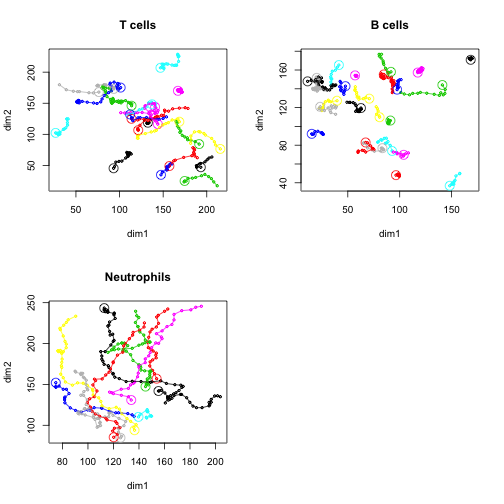We can also plot 3D tracks using the function plot3d() (which requires the package scatterplot3d): par( mfrow = c(2,2) ) plot3d( TCells, main = "T cells" ) plot3d( BCells, main = "B cells" ) plot3d( Neutrophils, main = "Neutrophils" )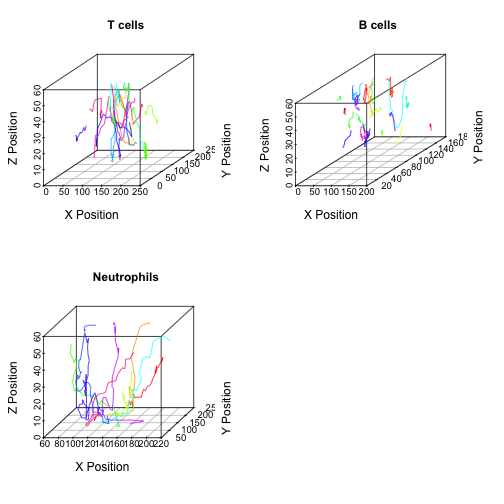### 1.2 Star plots Finally, we can overlay the track starting points using normalizeTracks() – which is useful to detect directionality: par( mfrow = c(2,2) ) plot( normalizeTracks(TCells), main = "T cells" ) plot( normalizeTracks(BCells), main = "B cells" ) plot( normalizeTracks(Neutrophils), main = "Neutrophils" )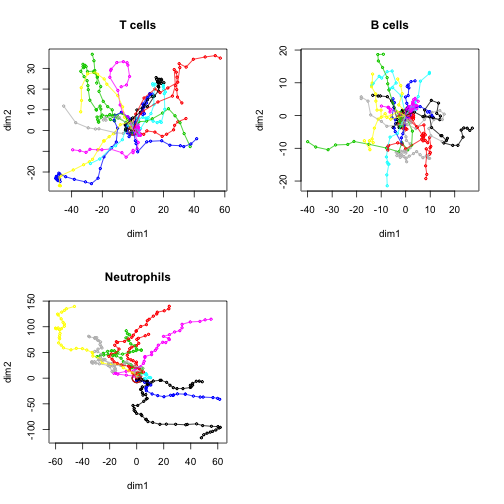# 2 Quantification of measures on tracks The package contains several measures that can be computed on single tracks (see ?TrackMeasures for an overview). The following sections will give examples on how to compute these metrics in different ways, using the speed() function as an example. ### 2.1 Cell-based analyses To compute a metric for each track in a dataset, use R's sapply() function directly on the tracks object: # Obtain mean speeds for each track using sapply Tcell.speeds <- sapply( TCells, speed ) str( Tcell.speeds )  ## Named num [1:22] 0.0411 0.1641 0.1465 0.1283 0.0859 ... ## - attr(*, "names")= chr [1:22] "0" "1" "2" "3" ...  summary( Tcell.speeds )  ## Min. 1st Qu. Median Mean 3rd Qu. Max. ## 0.04112 0.13284 0.17977 0.17955 0.22692 0.34978  This returns a mean speed for each of the 22 tracks in the TCell dataset. Visualize the distribution: hist( Tcell.speeds )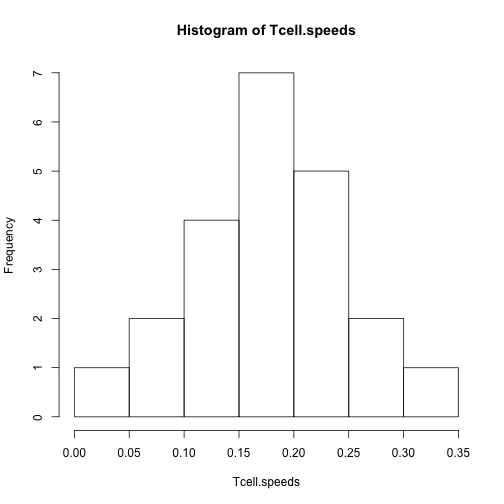Now let's repeat for the other two celltypes and plot them next to each other for comparison Bcell.speeds <- sapply( BCells, speed ) Nphil.speeds <- sapply( Neutrophils, speed ) # Create a dataframe of all data dT <- data.frame( cells = "T cells", speed = Tcell.speeds ) dB <- data.frame( cells = "B cells", speed = Bcell.speeds ) dN <- data.frame( cells = "Neutrophils", speed = Nphil.speeds ) d <- rbind( dT, dB, dN ) # Compare: ggplot( d, aes( x = cells, y = speed ) ) + ggbeeswarm::geom_quasirandom() + scale_y_continuous( limits = c(0,NA) ) + theme_classic()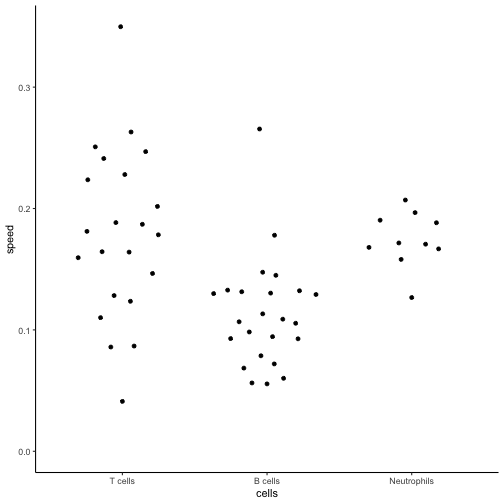### 2.2 Step-based analyses It has been suggested that cell-based analyses introduce biases, and that analyses should be performed on individual “steps” from all tracks combined instead (Beltman et al (2009)). We can extract such steps using the subtracks() function (where i is the subtrack length, which is 1 for a single step): Tcell.steps <- subtracks( TCells, i = 1 )  We can then proceed as before to obtain speeds for each step and further summary statistics: # Obtain instantaneous speeds for each step using sapply Tcell.step.speeds <- sapply( Tcell.steps, speed ) str( Tcell.step.speeds )  ## Named num [1:359] 0.05 0.1207 0.0509 0.0587 0.0264 ... ## - attr(*, "names")= chr [1:359] "0.1" "0.2" "0.3" "0.4" ...  summary( Tcell.step.speeds )  ## Min. 1st Qu. Median Mean 3rd Qu. Max. ## 0.007591 0.073433 0.144298 0.159449 0.226024 0.664722  Visualize the distribution: hist( Tcell.step.speeds )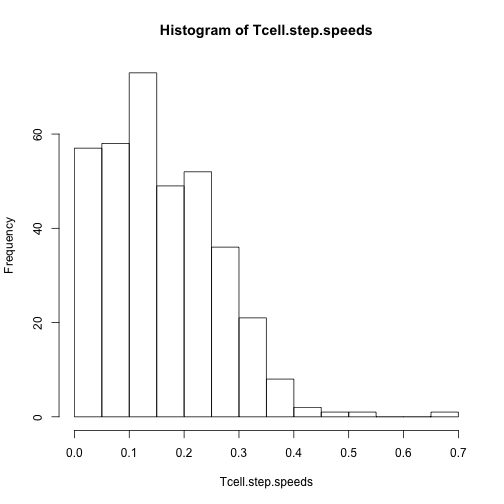Compare cell-based versus step-based: d.step <- data.frame( method = "step-based", speed = Tcell.step.speeds ) d.cell <- data.frame( method = "cell-based", speed = Tcell.speeds ) d.method <- rbind( d.step, d.cell ) ggplot( d.method, aes( x = method, y = speed ) ) + ggbeeswarm::geom_quasirandom( size = 0.3 ) + scale_y_continuous( limits = c(0,NA) ) + theme_classic()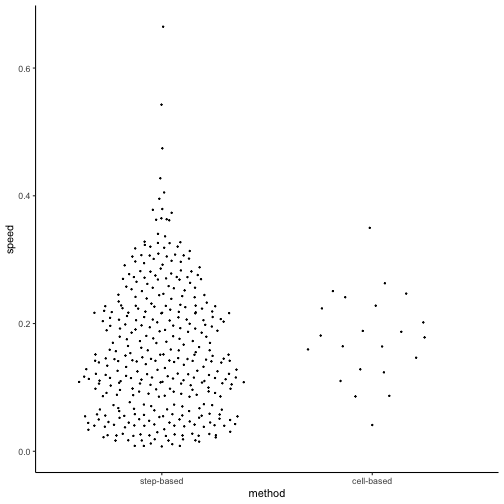And again compare between the different celltypes: Bcell.step.speeds <- sapply( subtracks(BCells,1), speed ) Nphil.step.speeds <- sapply( subtracks(Neutrophils,1), speed ) # Create a dataframe of all data dT <- data.frame( cells = "T cells", speed = Tcell.step.speeds ) dB <- data.frame( cells = "B cells", speed = Bcell.step.speeds ) dN <- data.frame( cells = "Neutrophils", speed = Nphil.step.speeds ) dstep <- rbind( dT, dB, dN ) # Compare: ggplot( dstep, aes( x = cells, y = speed ) ) + ggbeeswarm::geom_quasirandom( size = 0.3 ) + scale_y_continuous( limits = c(0,NA) ) + theme_classic()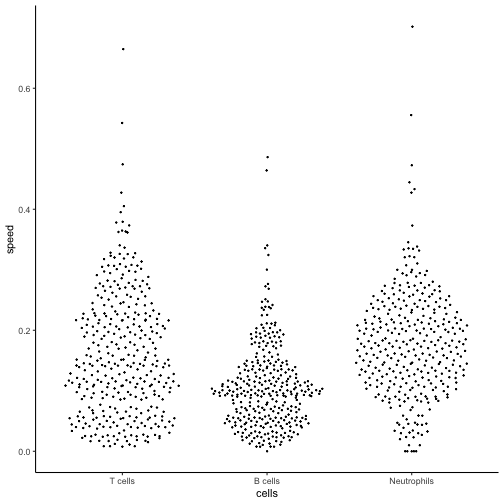Note that we can do something similar for turning angles, but we need at least two steps to compute a turning angle. Therefore, use subtracks() with i=2 in combination with the overallAngle() method: Tcell.step.angle <- sapply( subtracks(TCells,2), overallAngle ) Bcell.step.angle <- sapply( subtracks(BCells,2), overallAngle ) Nphil.step.angle <- sapply( subtracks(Neutrophils,2), overallAngle ) # Create a dataframe of all data dT <- data.frame( cells = "T cells", turn.angle = Tcell.step.angle ) dB <- data.frame( cells = "B cells", turn.angle = Bcell.step.angle ) dN <- data.frame( cells = "Neutrophils", turn.angle = Nphil.step.angle ) dangle <- rbind( dT, dB, dN ) # convert radians to degrees dangle$turn.angle <- pracma::rad2deg( dangle$turn.angle ) # Compare: ggplot( dangle, aes( x = cells, y = turn.angle ) ) + ggbeeswarm::geom_quasirandom( size = 0.3 ) + scale_y_continuous( limits = c(0,NA) ) + theme_classic()  ## Warning: Removed 12 rows containing missing values (position_quasirandom).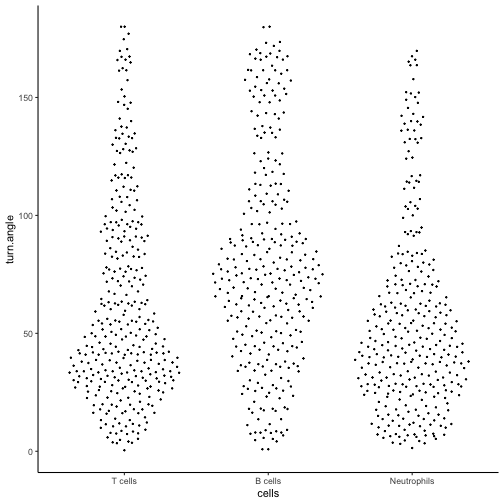### 2.3 Staggered analyses Another approach is to compute metrics not only on “steps” (subtracks of length one), but on all possible subtracks in a track. This is called a “staggered” analysis (Mohktari et al (2013)). To see how this works on a single track, use applyStaggered with matrix=TRUE. For example, consider track number 8 in the TCells data, which has 8 coordinates (7 steps): x <- TCells[] x  ## t x y z ## 1 55.484 76.2741 167.950 41.25000 ## 2 83.296 69.9965 166.565 31.25000 ## 3 111.093 64.8542 166.904 23.75000 ## 4 138.906 54.0290 168.794 21.25000 ## 5 166.656 51.8075 168.686 18.75000 ## 6 194.406 47.0689 169.251 16.25000 ## 7 222.078 38.9196 171.741 16.25000 ## 8 249.890 31.0750 179.754 1.55196  If we compute the speed on all staggered subtracks, we get the following matrix: stag <- applyStaggered( x, speed, matrix = TRUE ) stag  ## [,1] [,2] [,3] [,4] [,5] [,6] [,7] ## [1,] NA 0.4274444 0.3774206 0.3866812 0.3202594 0.2950665 0.2972042 ## [2,] 0.4274444 NA 0.3273697 0.3662945 0.2844984 0.2619309 0.2711040 ## [3,] 0.3774206 0.3273697 NA 0.4051969 0.2630508 0.2400975 0.2570119 ## [4,] 0.3866812 0.3662945 0.4051969 NA 0.1205820 0.1573604 0.2074583 ## [5,] 0.3202594 0.2844984 0.2630508 0.1205820 NA 0.1941387 0.2509575 ## [6,] 0.2950665 0.2619309 0.2400975 0.1573604 0.1941387 NA 0.3079365 ## [7,] 0.2972042 0.2711040 0.2570119 0.2074583 0.2509575 0.3079365 NA ## [8,] 0.3497818 0.3368165 0.3387084 0.3220461 0.3892137 0.4867794 0.6647221 ## [,8] ## [1,] 0.3497818 ## [2,] 0.3368165 ## [3,] 0.3387084 ## [4,] 0.3220461 ## [5,] 0.3892137 ## [6,] 0.4867794 ## [7,] 0.6647221 ## [8,] NA  Where point $$(i,j)$$ contains the mean speed in the subtrack from the $$i^{th}$$ to the $$j^{th}$$ coordinate (thus, the diagonal contains subtracks from a coordinate to itself – which is no subtrack at all but a single coordinate, and therefore has no speed). Visualize this using the image() or filled.contour() functions: image( stag )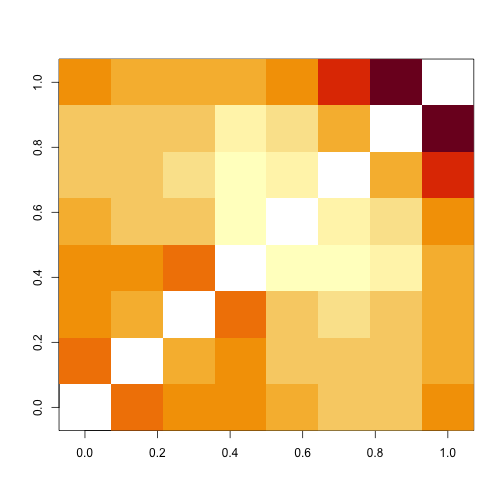filled.contour( stag )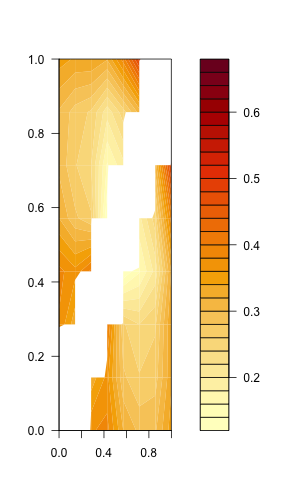Now do it again for a longer track: filled.contour( applyStaggered( TCells[], speed, matrix = TRUE ) )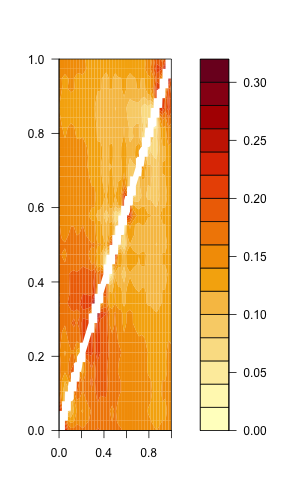This nicely illustrates fluctuations in speed at different points in the track. If we leave out the matrix=TRUE, the function simply returns the mean of all non-NA entries in the matrix: # These are the same: applyStaggered( TCells[], speed )  ##  0.3181119  mean( stag, na.rm = TRUE )  ##  0.3181119  applyStaggered only works on a single track, not a tracks object. To directly get the staggered means for all tracks in the object, use the staggered option: # Compare the three different methods Tcell.stag.speed <- sapply( TCells, staggered( speed ) ) d.staggered <- data.frame( method = "staggered", speed = Tcell.speeds ) d.method <- rbind( d.step, d.cell, d.staggered ) ggplot( d.method, aes( x = method, y = speed ) ) + ggbeeswarm::geom_quasirandom( size = 0.3 ) + scale_y_continuous( limits = c(0,NA) ) + theme_classic()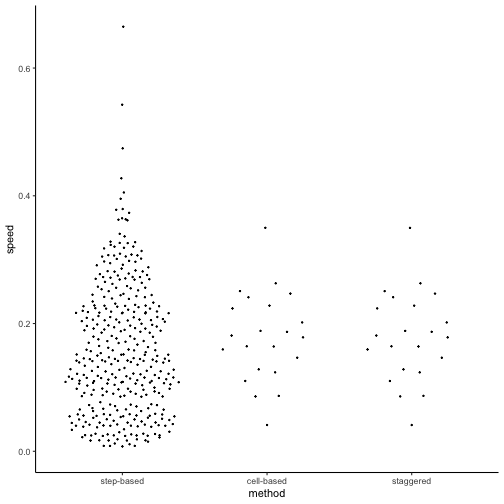# cell-based and staggered cell-based are slightly different: hist( Tcell.speeds - Tcell.stag.speed )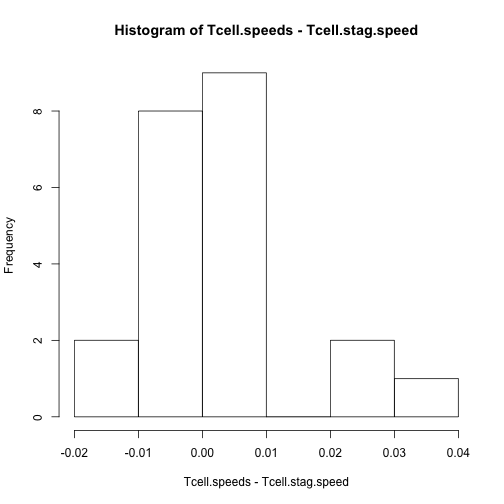# 3 Mean square displacement plots A common analysis on migration data is generating mean square displacement (MSD) plots. For a range of time interval $$\Delta t$$, we compute the average squared displacement of all subtracks with that duration. Like with step-based approaches, we compute the metric on subtracks pooled from all individual cells – but now we also do this for subtracks of more than one step. We can use the subtracks() function with varying i argument to obtain subtracks of different lengths, and then use sapply() to find a mean metric for each of these datasets. However, the package also contains a convenience function that does this automatically: aggregate(). For example, use this function to directly get the mean square displacement of all single steps: aggregate( TCells, squareDisplacement, subtrack.length = 1 )  ## i value ## 1 1 27.67111  where “i” is the subtrack length in number of steps, and “value” is the average value of the applied metric (in this case, square displacement) for all subtracks of that length in the data. We can also report different summary statistics by changing the FUN argument (see ?aggregate.tracks): aggregate( TCells, squareDisplacement, subtrack.length = 1, FUN = "mean.se" )  ## i mean lower upper ## 1 1 27.67111 25.86344 29.47878  This gives us not only the mean, but also the standard error. Now, if we leave out the subtrack.length, aggregate() automatically computes the metric for subtracks of all possible lengths in the dataset and returns those in a dataframe: Tcell.msd <- aggregate( TCells, squareDisplacement, FUN = "mean.se" ) str( Tcell.msd )  ## 'data.frame': 38 obs. of 4 variables: ##$ i    : num  1 2 3 4 5 6 7 8 9 10 ...
##  $mean : num 27.7 81.6 154.8 239.5 329.2 ... ##$ lower: num  25.9 76.9 146.1 225.8 309.4 ...
##  $upper: num 29.5 86.2 163.6 253.2 349 ...  We can then use this for plotting: ggplot( Tcell.msd, aes( x = i, y = mean ) ) + geom_ribbon( aes( ymin = lower, ymax = upper) , alpha = 0.2 ,color=NA ) + geom_line( ) + labs( x = expression( paste(Delta,"t (steps)") ), y = "mean square displacement") + theme_classic()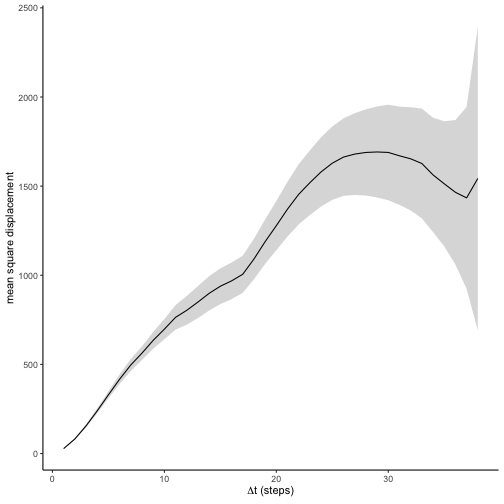Plotting the standard error is useful because, as $$\Delta t$$ increases, the number of subtracks with that length decreases rapidly. MSD values at the high end of the plot therefore tend to be less reliable as they are based on very few observations. Compare for example the number of subtracks of length 10 to the number of length 35: num10 <- length( subtracks( TCells, 10 ) ) num35 <- length( subtracks( TCells, 35 ) ) c( "10" = num10, "35" = num35 )  ## 10 35 ## 164 12  Now, let's compare the MSD plot for the three different types of cells: # Combine into a single dataframe with one column indicating the celltype # To truly compare them, report subtrack length not in number of steps but # in their duration (which may differ between different datasets) Tcell.msd$cells <- "T cells"
Tcell.msd$dt <- Tcell.msd$i * timeStep( TCells )
Bcell.msd <- aggregate( BCells, squareDisplacement, FUN = "mean.se" )
Bcell.msd$cells <- "B cells" Bcell.msd$dt <- Bcell.msd$i * timeStep( BCells ) Nphil.msd <- aggregate( Neutrophils, squareDisplacement, FUN = "mean.se" ) Nphil.msd$cells <- "Neutrophils"
Nphil.msd$dt <- Nphil.msd$i * timeStep( Neutrophils )
msddata <- rbind( Tcell.msd, Bcell.msd, Nphil.msd )

##   i      mean     lower     upper   cells        dt
## 1 1  27.67111  25.86344  29.47878 T cells  27.79700
## 2 2  81.55090  76.90564  86.19616 T cells  55.59399
## 3 3 154.84639 146.13937 163.55341 T cells  83.39099
## 4 4 239.48300 225.78660 253.17940 T cells 111.18799
## 5 5 329.17890 309.39604 348.96176 T cells 138.98499
## 6 6 416.84370 389.97834 443.70905 T cells 166.78198

# Plot
p1 <- ggplot( msddata, aes( x = dt , y = mean, color = cells, fill = cells ) ) +
geom_ribbon( aes( ymin = lower, ymax = upper) , alpha = 0.2 ,color=NA ) +
geom_line( ) +
labs( x = expression( paste(Delta,"t (seconds)") ),
y = "mean square displacement") +
theme_classic()
# Also make a zoomed version to look only at first part of the plot
pzoom <- p1 + coord_cartesian( xlim = c(0,500), ylim = c(0,5000) )
gridExtra::grid.arrange( p1, pzoom, ncol = 2 )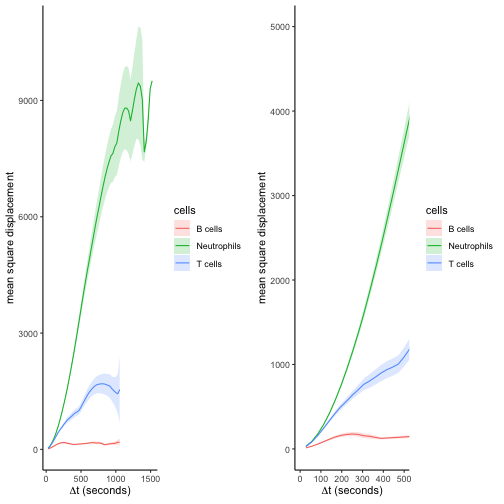Note that aggregate() can also make MSD plots from non-overlapping subtracks or from subtracks starting at the first position of each track; see ?aggregate.tracks for details.

# 4 Analyzing persistence

The downside of the MSD plot is that it is strongly affected by both speed and directionality of cells: faster cells displace more, as do cells that move straighter. It can therefore be worthwile to study cell speed and straightness or “persistence” independently.

### 4.1 Straightness metrics

The package implements several metrics that provide a measure of track straightness or directionality (see Mohktari et al (2013) for details): displacementRatio(), outreachRatio, straightness(), and asphericity(). These metrics can be applied to the data in the same way as was done for speed() in section 2 of this tutorial. See also ?TrackMeasures for more information.

### 4.2 Autocorrelation plots

To analyze cell persistence, another good analysis is the autocorrelation plot: taking steps with $$\Delta t$$ time in between, how much do their directions of movement still correlate?

Officially, the autocorrelation plot is a plot of $$\cos \alpha$$ of two steps, $$\vec{s_1}$$ and $$\vec{s_2}$$, whose starting points are $$\Delta t$$ apart. This is equal to the dot product between $$\vec{s_1}$$ and $$\vec{s_2}$$ after normalizing both vectors to unit length. We can compute this again using aggregate() (see also the previous section):

Tcell.acor <- aggregate( TCells, overallNormDot, FUN = "mean.se"  )
Tcell.acor$dt <- Tcell.acor$i * timeStep(TCells)
Tcell.acor$cells <- "T cells" ggplot( Tcell.acor, aes( x = dt , y = mean, color = cells, fill = cells ) ) + geom_hline( yintercept = 0 ) + geom_ribbon( aes( ymin = lower, ymax = upper) , alpha = 0.2 ,color=NA ) + geom_line( ) + labs( x = expression( paste(Delta,"t (seconds)") ), y = expression(cos(alpha))) + theme_classic() + theme( axis.line.x = element_blank() )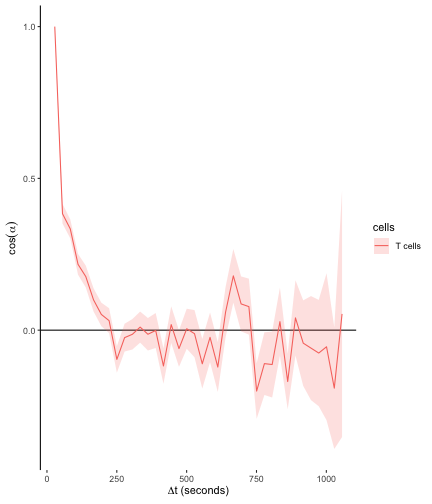Note, however, that steps in which the cell barely moves can add a lot of noise to the analysis of angles. Since it makes no sense anyway to speak of persistent movement when a cell is not moving, we can avoid this by filtering for pairs of steps $$\vec{s_1}$$ and $$\vec{s_2}$$ that have at least some minimum displacement. To do this, we give aggregate() a function in the filter.subtracks argument: # Returns TRUE if first and last step of a track are of the minimum length of 1 micron check <- function(x){ all( sapply( list(head(x,2),tail(x,2)), trackLength ) >= 1.0 ) } # repeat analysis Tcell.acor2 <- aggregate( TCells, overallNormDot, FUN = "mean.se", filter.subtracks = check ) Tcell.acor2$dt <- Tcell.acor2$i * timeStep(TCells) Tcell.acor2$cells <- "T cells (filtered)"

d <- rbind( Tcell.acor, Tcell.acor2 )

# compare
ggplot( d, aes( x = dt , y = mean, color = cells, fill = cells ) ) +
geom_hline( yintercept = 0 ) +
geom_line( ) +
labs( x = expression( paste(Delta,"t (seconds)") ),
y = expression(cos(alpha))) +
theme_classic() +
theme( axis.line.x = element_blank() )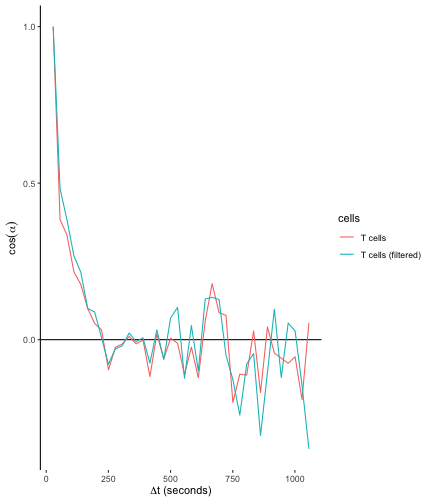The drop in autocorrelation is now slightly less steep, because the lack of correlation between steps where the cell does not move is not taken into account.

Another solution is to make the autocovariance plot instead of the autocorrelation plot, where we plot the dot product between the original unnormalized vectors $$\vec{s_1} \bullet \vec{s_2}$$ instead of normalizing them fist. The dot product is less sensitive to noise from very short steps and is therefore recommended:

# autocovariance
Tcell.acov <- aggregate( TCells, overallDot,
FUN = "mean.se" )
Tcell.acov$dt <- Tcell.acov$i * timeStep(TCells)
Tcell.acov$cells <- "T cells" # compare ggplot( Tcell.acov, aes( x = dt , y = mean, color = cells, fill = cells ) ) + geom_hline( yintercept = 0 ) + geom_line( ) + labs( x = expression( paste(Delta,"t (seconds)") ), y = "autocovariance" ) + theme_classic() + theme( axis.line.x = element_blank() )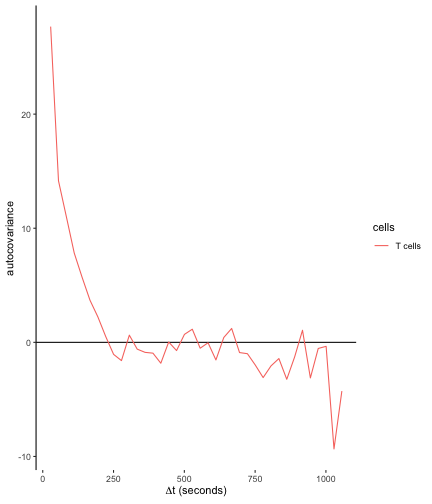However, the autocovariance is no longer a number between 0 and 1, which is somewhat less convenient when comparing cell types moving at different speeds. We therefore divide all values by the maximum autocovariance at $$\Delta t = 0$$, and compare with the other celltypes: # Normalized autocovariance Tcell.acov[,2:4] <- Tcell.acov[,2:4] / Tcell.acov$mean

# Other cells
Bcell.acov <- aggregate( BCells, overallDot, FUN = "mean.se" )
Bcell.acov$cells <- "B cells" Bcell.acov$dt <- Bcell.acov$i * timeStep( BCells ) Bcell.acov[,2:4] <- Bcell.acov[,2:4] / Bcell.acov$mean

Nphil.acov <- aggregate( Neutrophils, overallDot, FUN = "mean.se" )
Nphil.acov$cells <- "Neutrophils" Nphil.acov$dt <- Nphil.acov$i * timeStep( Neutrophils ) Nphil.acov[,2:4] <- Nphil.acov[,2:4] / Nphil.acov$mean

acovdata <- rbind( Tcell.acov, Bcell.acov, Nphil.acov )

# compare
p1 <- ggplot( acovdata, aes( x = dt , y = mean, color = cells, fill = cells ) ) +
geom_hline( yintercept = 0 ) +
geom_ribbon( aes( ymin = lower, ymax = upper) , alpha = 0.2 ,color=NA ) +
geom_line( ) +
labs( x = expression( paste(Delta,"t (seconds)") ),
y = "autocovariance" ) +
theme_classic() +
theme( axis.line.x = element_blank() )

pzoom <- p1 + coord_cartesian( xlim = c(0,500) )
gridExtra::grid.arrange( p1, pzoom, ncol = 2 )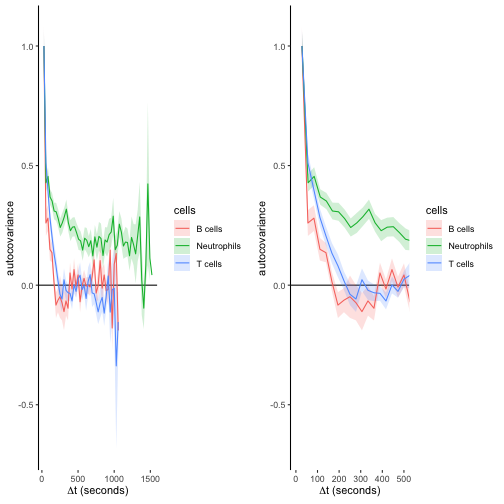B cells lose their autocovariance most rapidly, making them the least persistent. Neutrophils maintain directional persistence over longer timescales.

# 5 Analyzing directionality

Aside from directionality within a track segment (because of directional persistence), there can also be global directionality between different tracks: for example, when cells are moving in the direction of some target. The package supports several types of analyses to detect such directionality in a dataset.

### 5.1 Hotelling's test

An unbiased method for detecting global directionality is Hotelling's T-square test (see Textor et al (2011)), which tests if the mean step displacement vector is significantly different from the null vector. This method is powerful for detecting global movement biases when the direction is not known in advance.

For example, applying Hotelling's test to the Neutrophil data:

par( mfrow=c(1,2) )
plot( Neutrophils )
hotellingsTest( Neutrophils, plot = TRUE, step.spacing = 3 )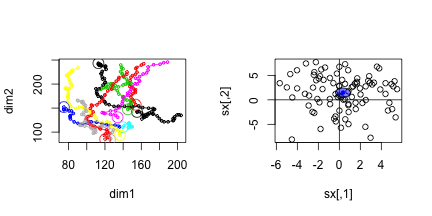##
##  Hotelling's one sample T2-test
##
## data:
## T2 = 17.457, df1 = 2, df2 = 88, p-value = 0.000378
## alternative hypothesis: true location is not equal to c(0,0)


This shows there is a bias towards movement in the positive $$y$$ direction. See the discussion of tissue drift in the vignette on quality control for a more extensive discussion on how to use Hotelling's test to detect a global directional bias.

### 5.2 Angle analyses

#### 5.2.1 Overview: Angles and distances of steps to a fixed reference (point, plane, or direction)

The following functions support the computation of angles and distances of steps compared to a fixed reference:

• angleToPoint( x, from = 1, p = c(1,1,1) ) : angle to the reference point with coordinates p.
• angleToDir( x, from = 1, dvec = c(1,1,1) ) : angle to a reference direction specified by vector dvec.
• angleToPlane( x, from = 1, p1, p2, p3 ) : angle to a plane specified by the points p1, p2, and p3.
• distanceToPlane( x, from = 1, p1, p2, p3 ) : distance to a plane specified by the points p1, p2, and p3.
• distanceToPoint( x, from = 1, p ) : distance to the reference point with coordinates p.

These functions take each track in tracks object x, extract the single steps starting at index from (by default, this is the first step in the track), and compute the angle of this step towards the reference. The distance functions compute the distance of the step starting point to the reference.

#### 5.2.2 Angles and distances to planes: detecting tracking artefacts

Angles and distances in reference to a plane are useful to detect tracking artefacts, see the vignette on quality control for a detailed example.

#### 5.2.3 Angle to a reference direction: powerful directionality tests when direction is known

While Hotelling's test may be used to test for directionality in an unknown direction, we can increase the power of testing when the direction is known (for example, in an experiment with an artificial chemotactic gradient in a known direction).

As an example, let's make an artificial dataset with additional movement in the direction $$(1,1,1)$$:

# Directional movement is 0.05 micron/sec in each dimension
directional.speed <- c( 0.05, 0.05, 0.05 )
add.dir <- function( x, speed.vector )
{
# separate timepoints and coordinates
tvec <- x[,1]
coords <- x[,-1]

# compute movement due to drift.
speed.matrix <- matrix( rep( speed.vector, nrow(coords) ),
ncol = ncol(coords), byrow= TRUE )
speed.matrix <- speed.matrix * tvec